## Thursday, 18 November 2010

### Plus or Minus InfinityCame across the problem above at the Math Central web page from Canada. I had written before about the values of iterated roots, but had not considered the case of what happens if they are joined by subtracting instead of adding. It turns out that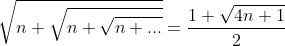. The derivation of this can be found in a short paper I wrote here.

By a similar approach we see that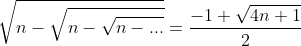making the problem above pretty simple as an aftermath of all this... but I wondered if there was an intuitive approach to justify this without the actual itereation results....nothing jumps out yet....

It also made me wonder about alternating signs...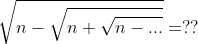.

Playing around with this I came up with a fourth degree equation to solve, with two positive roots...Yikes, but one of them was a spurious solution created by a second squaring of the equation. When I worked it out for n=2, I got a real surprise.. it led me back to the itereated sequence which started me on this exploration way back when...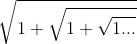... it turns out that the iteration of roots of two using alternating signs is deeply related to the iteration of roots of one using addition. I'll leave it to you to explore...

And their difference, if I have calculated correctly, is the iterated square roots of 3 using alternating signs.... Too much to explore,...... more later, but I just figured out in class today (I hope) that the series with alternating signs will equal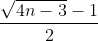and produces an integer value when the value of n is =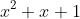for integer x.

Taylor said...

Everytime I've seen this infinite radical written out, I've always plugged n=0 into the equation which results in an answer of 1! Any quick explanation of why that might be?

Oh, love the blog. Was pointed to it today and have been reading back over older articles.

Pat's Blog said...

Taylor,
Thanks for the kind comments... I'm not sure I caught which infinite radical you mean.... or why you get one... help me see what you mean and maybe I can be more help...

Taylor said...

Hey Pat,

Sorry for the ambiguity.

Say you have a radical like the second image in this post:

sqrt(n+sqrt(n+sqrt(n+sqrt(n+...)))

You've shown that this is equal to [1 + sqrt(4n+1)]/2

Now, say you let n=0, then the infinite radical is then equal to [1 + sqrt(4n+1)]/2 = [1 + sqrt(4(0)+1)]/2 = [1 + sqrt(1)]/2 = [1+1]/2 = 1

Hope that helps!

Taylor said...

Looking at the solution a little harder now, it seems it stems from the fact we're using the quadratic formula for the solution to the infinite radical.

The infinite radical actually has 2 solutions: [1 + sqrt(4n+1)]/2 AND [1 - sqrt(4n+1)]/2. That second solution gives 0 when n=0, which is what I would expect, however there is still this other solution of 1 that is very puzzling. Your thoughts are appreciated.

Pat's Blog said...

Ok, I think I understand.... The derivation goes like this:
if the value of the constant was a and the sum of the iteration is N, then N^2 = a +N....

I presumed that a>0, but if it was equal to zero, then we have N^2 = N, which is only true when N=0 or N=1
Hope that helps.